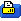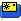# Control Tower - December 1998

### Part 4 of "Understanding Electric Power Systems".

Understanding Electric Power Systems - Part 4

This month I am presenting the fourth part in my series on electric power systems.

Recap

In the November column I presented a simplified formula for computing the power absorbed by a propeller of any given diameter and pitch.  I also provided some rules of thumb to help explain the interaction of variables such as pitch, current draw, voltage, etc.

Last month's Q&A was very challenging.  I am providing complete, detailed answers to all of the questions below.  Strive to understand the explanations I have provided, even if you did not complete the Q&A on your own.

In order to learn things, the human mind requires practice.  Not everyone is interested enough to work through these complex problems, but those that do will be rewarded with a much better recollection of the material.  Depending on your enthusiasm about the hobby, you may find these kind of problems very rewarding.  If not, then try to at least absorb the summary information at the end of each column.

Moving into the Real World

Our Ideal Motors and Cells have been useful tools that serve as great illustrations, but they fall short of thoroughly representing the real world.  In the real world we cannot convert electrical energy into mechanical energy with 100% efficiency.  Some of the energy will be "lost" as heat (though energy is never really lost per se, we might as well think of it that way because the heat we generate is of no use to us).

If we set out to create a perfectly accurate model of an electric power system, we would soon be in over our heads in numbers.  Instead, we must compromise by selecting a few very important parameters which account for the majority of the losses we might encounter.  We will end up with an understanding that is far better than the Ideal model but only a little more complex.

Before we go any further, we must have a basic understanding of electricity.

Resistance, and Ohms Law

Electrons are not able to pass through a conductor without encountering some friction along the way.  The amount of friction produced by the conductor is called resistance.

Resistance is measured in Ohms, and its relationship to voltage and current is summed up in Ohm's Law:

I = V / R

In this formula, "I" represents current, "V" represents voltage, and "R" represents resistance.  One Ohm is equal to one volt per amp.

What Ohm's Law is saying is that the voltage and resistance of a circuit are what determines the current.  This is because "current" is an expression of how fast energy is moving from one side of a circuit to another.  If the resistance of a circuit is very low the energy can move across the circuit very quickly, which means the current is high.  If the resistance is very high then the energy cannot move quickly.   Instead, it must be pushed very hard to make it across the circuit.  A circuit with a lot of resistance generates much more heat and wastes a lot more energy than a circuit without a lot of resistance.

We can rework Ohm's law so that we can solve for voltage or resistance if necessary:

V = IR
R = V/I

And we can also rework our Power formula using Ohm's Law so that we can calculate power using any two of the three quantities:

Power = Volts x Amps = V x I
Power = V x I = IR x I = I^2 x R
Power = V x I = V x V/R = V^2 / R

The Water and Pipes Analogy

A very common way of explaining Ohm's law and electricity in general is to use an analogy involving a bucket of water and a pipe.

Imagine that you have a bucket of a certain size which is on a pedestal of a certain height.  A pipe is attached to the bottom of the bucket.  Water is able to flow through the pipe until the bucket is completely depleted of liquid.

In our analogy we consider the bucket to be a battery, the height of the pedestal to be voltage, and the rate of the water as it travels through the pipe to be current.

If we raise up the pedestal (increase the voltage), the water will travel down the pipe with more force, just as the electrons will travel across a conductor with more force if the voltage is increased.  If we increase the size of the bucket we will have more water, akin to increasing a battery's capacity.  If we make our pipe a bigger diameter or provide a very smooth pipe in place of a rough one, the water will travel down the pipe quicker, much like lowering the resistance of an electrical circuit.

The total amount of water coming out of the pipe over a given second would be like the amount of power going through a power system in a given second.  A large diameter pipe and a small pedestal would let us get a lot of water very slowly while a really tall pedestal and a narrow pipe would let us get a little bit of water very quickly.  Both setups could give us the same volume of water over a given period of time.  This is very similar to the relationship between volts and amps in regards to power.

Like most analogies, this one can be carried too far, but please refer to it when necessary.

The Big 4

We will expand our motor model to better reflect the real world by adding four different parameters:

1. Armature resistance
3. RPM limit
4. Torque limit

These parameters are all we need to make reasonably accurate predictions of power system performance.  This month we will discuss Armature Resistance.

Armature Resistance

A motor has electrical resistance mostly due to the coils of wire in the armature (or stator in a brushless motor).  This resistance works against the voltage supplied to the motor and has the effect of depressing the Kv of the motor.  Typically, armature resistance is abbreviated as Rm.

In the Ideal World, the RPM of a motor was determined by the following formula:

RPM = Kv x Voltage

In the Real World its a little more complex.  Recall Ohm's Law:

I = V / R

-or-

V = IR

If we take Ohm's law into account we can compute the effective voltage loss inside the motor.  This loss must be considered when predicting the RPM.  The loss is based on the armature resistance of the motor and the current draw.  In other words:

Vloss = I x Rm

So the greater the current the more the voltage is effectively lowered within the motor.  If we substitute everything out and solve for RPM we get:

RPM = Kv x V
RPM = Kv x (V - Vloss)
RPM = Kv x (V - I x Rm)

In English: the RPM of a motor is equal to its Kv times the input voltage minus losses due to armature resistance.  The losses due to armature resistance are computed as the input current multiplied by the armature resistance.

Lets imagine a motor with a Kv of 1000 and an armature resistance of .05 Ohms.   What will the RPM be if the power into the motor is 10 volts at 10 amps?

RPM = Kv x (V - I x Rm)
RPM = 1000 x (10 - 10 x .05)
RPM = 1000 x (10 - .5)
RPM = 9500

So at 10 amps we have lost 500 RPM from the ideal. What if we use a much larger propeller and run the same motor at 30 amps?  What will the RPM be then?

RPM =  Kv x (V - I x Rm)
RPM = 1000 x (10 - 30 x .05)
RPM = 8500

Note that this is a far cry from the simplified assumptions we have made so far in this series.  At 30 amps we have lost 1500 RPM from the ideal.  One can quickly see why it is important to have a low armature resistance in high current applications.   The Astro FAI 40 happens to have the same armature resistance as our example (.050 Ohms).  A high quality brushless motor that is designed for limited motor run applications might have an armature resistance below .010 Ohms.

In the real world, armature resistance goes up as the temperature of the armature increases.  This means that the RPM of a motor will actually drop over time, even given the exact same input voltage (this is in addition to any RPM drop you may already be aware of due to voltage sag of the batteries).  This is a complication that only sophisticated computer programs can attempt to model.

Stall Current

When we dealt with Ideal Motors the RPM was determined solely by the input voltage.   Now we know that, in the real world, the RPM will drop as the current (load) increases.  But what would happen if we were to provide a load so large that the motor could not turn at all?

If the motor shaft is held such that it cannot move at all then it is said to be in a stalled condition.  The motor will draw the maximum current possible from the battery and will most likely be destroyed very quickly.  The current that will be drawn in a stall condition is predicted using Ohm's Law:

Istall = Vin / Rm

Therefore, an Astro 40 FAI motor will attempt to draw roughly 200 amps if stalled while connected to a 10 volt power source.  Please note that the actual current will be far less due to the fact that none of the cells we use for electric flight are capable of sourcing 200 amps.

Summary:

• Ohm's law relates current to voltage and resistance in a circuit: I = V / R
• The RPM of a real-world motor can be expressed as: RPM = Kv x (V - I x R), where V is the input voltage to the motor, I is the current drawn by the motor, and R is the armature resistance.
• Power can be expressed as:  P = I^2* R  or  P = V^2/R in addition to P=VxI
• A motor is said to be stalled when its shaft is unable to turn.  When in a stalled condition, a motor will attempt to draw current as predicted by Ohm's law.  Istall = Vin / Rm

Q&A

• Suppose I have two motors with the same Kv and Rm.  Both of them are being run on 10 cells.  The first motor is spinning its propeller at 10,000 RPM.  The second motor is spinning its propeller at 9,500 RPM.  Which motor is spinning the larger propeller?
• Which motor will spin faster on 10 cells at 20 amps: a motor with a Kv of 1000 and an Rm of .050 Ohms or a motor with a Kv of 950 and an Rm of .010 Ohms?
• Joe is walking out to retrieve his Super-Thermal-2000 R/C electric sailplane at his local field when he accidentally bumps the throttle switch.  The propeller is unable to move.  He is using an 8 cell pack and a speed 600 motor with an Rm of .145 Ohms.   How much current will the motor attempt to draw in this stalled condition?

 Thread ToolsShow Printable VersionEmail this Page